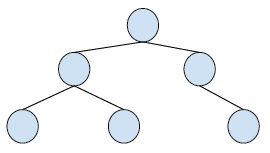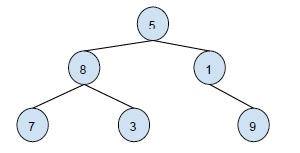# Print ancestors of a given binary tree node without recursion in C++

In this problem, we are given a binary tree and we have to print its ancestor of a node in a binary tree.

Binary Tree is a special tree whose every node has at max two child nodes. So, every node is either a leaf node or has one or two child nodes.

Example,The ancestor of a node in a binary tree is a node that is at the upper level of the given node.

Let’s take an example of ancestor node −Ancestors of a node with value 3 in this binary tree are 8,

For solving this problem, we will traverse from the root node to the target node. Step by step downwards in the binary tree. And in the path print all the nodes that come.

This will ideally involve the recursive calling of the same methods with each node that comes in the path from the root node to the target node.

So, a non-recursive approach will require the usage of iterative traversal and a stack that will store the ancestors of the target node in the tree. We will do the postorder traversal of the binary tree. And store the ancestors to the stack, and at last print the contents of the stack which will be the ancestors of the node.

## Example

Live Demo

#include <stdio.h>
#include <stdlib.h>
#define MAX_SIZE 100
struct Node{
int data;
struct Node *left, *right;
};
struct Stack{
int size;
int top;
struct Node* *array;
};
struct Node* insertNode(int data){
struct Node* node = (struct Node*) malloc(sizeof(struct Node));
node->data = data;
node->left = node->right = NULL;
return node;
}
struct Stack* createStack(int size){
struct Stack* stack = (struct Stack*) malloc(sizeof(struct Stack));
stack->size = size;
stack->top = -1;
stack->array = (struct Node**) malloc(stack->size * sizeof(struct Node*));
return stack;
}
int isFull(struct Stack* stack){
return ((stack->top + 1) == stack->size);
}
int isEmpty(struct Stack* stack){
return stack->top == -1;
}
void push(struct Stack* stack, struct Node* node){
if (isFull(stack))
return;
stack->array[++stack->top] = node;
}
struct Node* pop(struct Stack* stack){
if (isEmpty(stack))
return NULL;
return stack->array[stack->top--];
}
struct Node* peek(struct Stack* stack){
if (isEmpty(stack))
return NULL;
return stack->array[stack->top];
}
void AncestorNodes(struct Node *root, int key){
if (root == NULL) return;
struct Stack* stack = createStack(MAX_SIZE);
while (1){
while (root && root->data != key){
push(stack, root);
root = root->left;
}
if (root && root->data == key)
break;
if (peek(stack)->right == NULL){
root = pop(stack);
while (!isEmpty(stack) && peek(stack)->right == root)
root = pop(stack);
}
root = isEmpty(stack)? NULL: peek(stack)->right;
}
while (!isEmpty(stack))
printf("%d ", pop(stack)->data);
}
int main(){
struct Node* root = insertNode(15);
root->left = insertNode(10);
root->right = insertNode(25);
root->left->left = insertNode(5);
root->left->right = insertNode(12);
root->right->left = insertNode(20);
root->right->right = insertNode(27);
root->left->left->left = insertNode(1);
root->left->right->right = insertNode(14);
root->right->right->left = insertNode(17);
printf("The ancestors of the given node are : ");
AncestorNodes(root, 17);
getchar();
return 0;
}

## Output

The ancestors of the given node are : 27 25 15